# Addition 3-digit and 2-digit numbers | without Regrouping | Maths Grade2

### Addition 3-digit and 2-digit numbers without Regrouping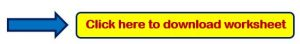Example 1:- Add the following?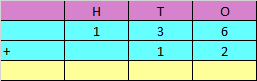Explanation:

Step 1: Adding the digits at one’s place,

6 + 2 = 8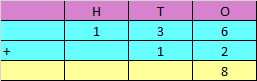Step 2: Adding the digits at ten’s place,

3 + 1 = 4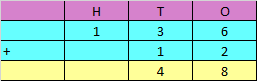Step 3: Adding the digits at hundred’s place,

1 + 0 = 1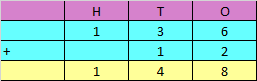Hence, the sum of the given numbers is 148

Example 2:- Add the following?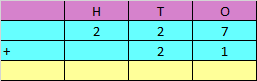Explanation:

Step 1: Adding the digits at one’s place,

7 + 1 = 8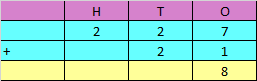Step 2: Adding the digits at ten’s place,

2 + 2 = 4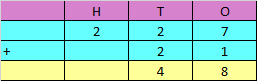Step 3: Adding the digits at hundred’s place,

2 + 0 = 2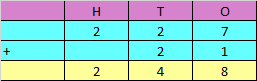Hence, the sum of the given numbers is 248

Example 3:- Add the following?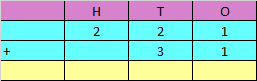Explanation:

Step 1: Adding the digits at one’s place,

1 + 1 = 2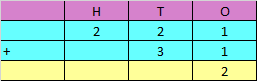Step 2: Adding the digits at ten’s place,

2 + 3 = 5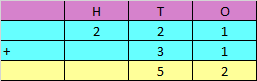Step 3: Adding the digits at hundred’s place,

2 + 0 = 2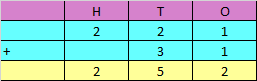Hence, the sum of the given numbers is 252